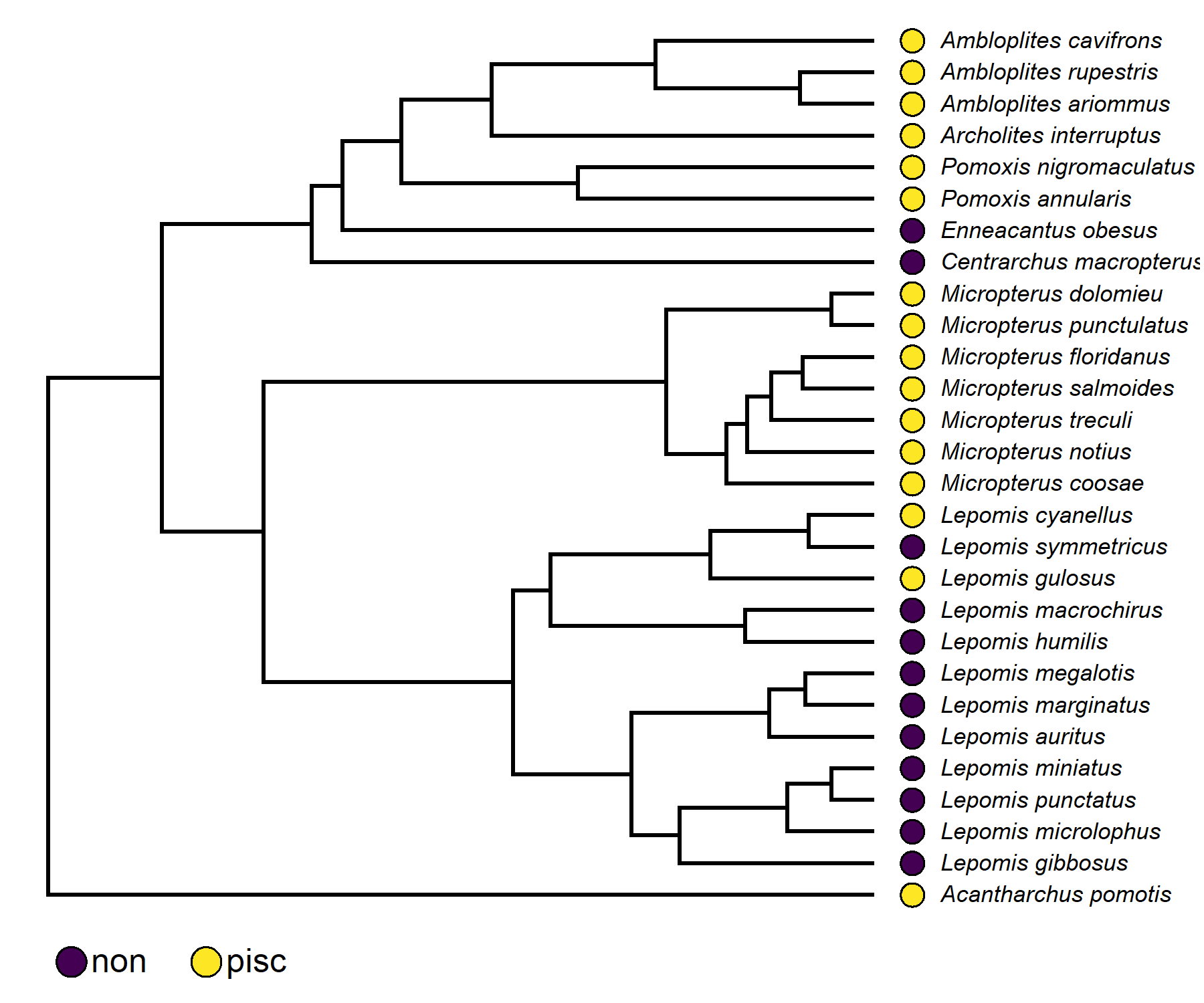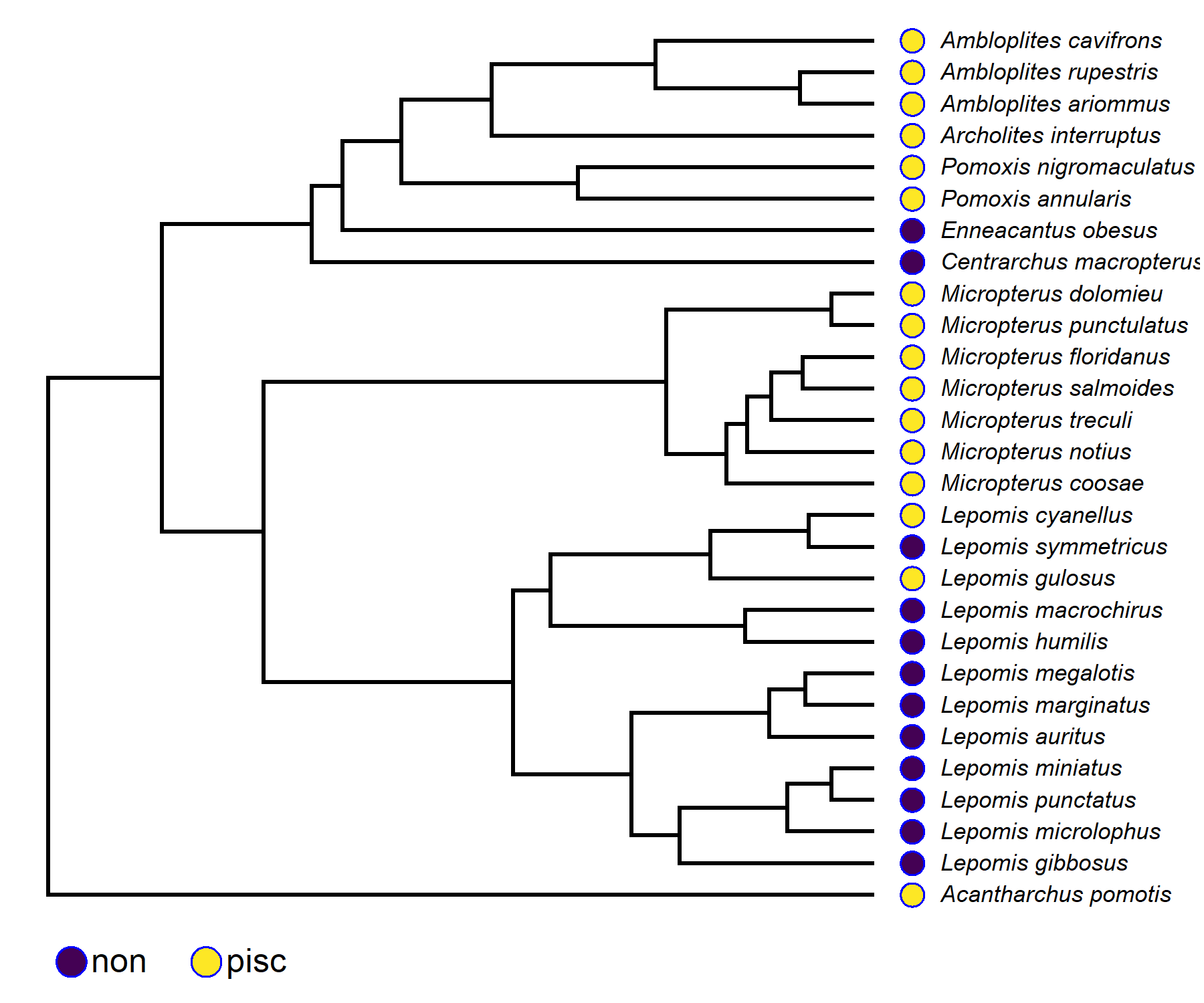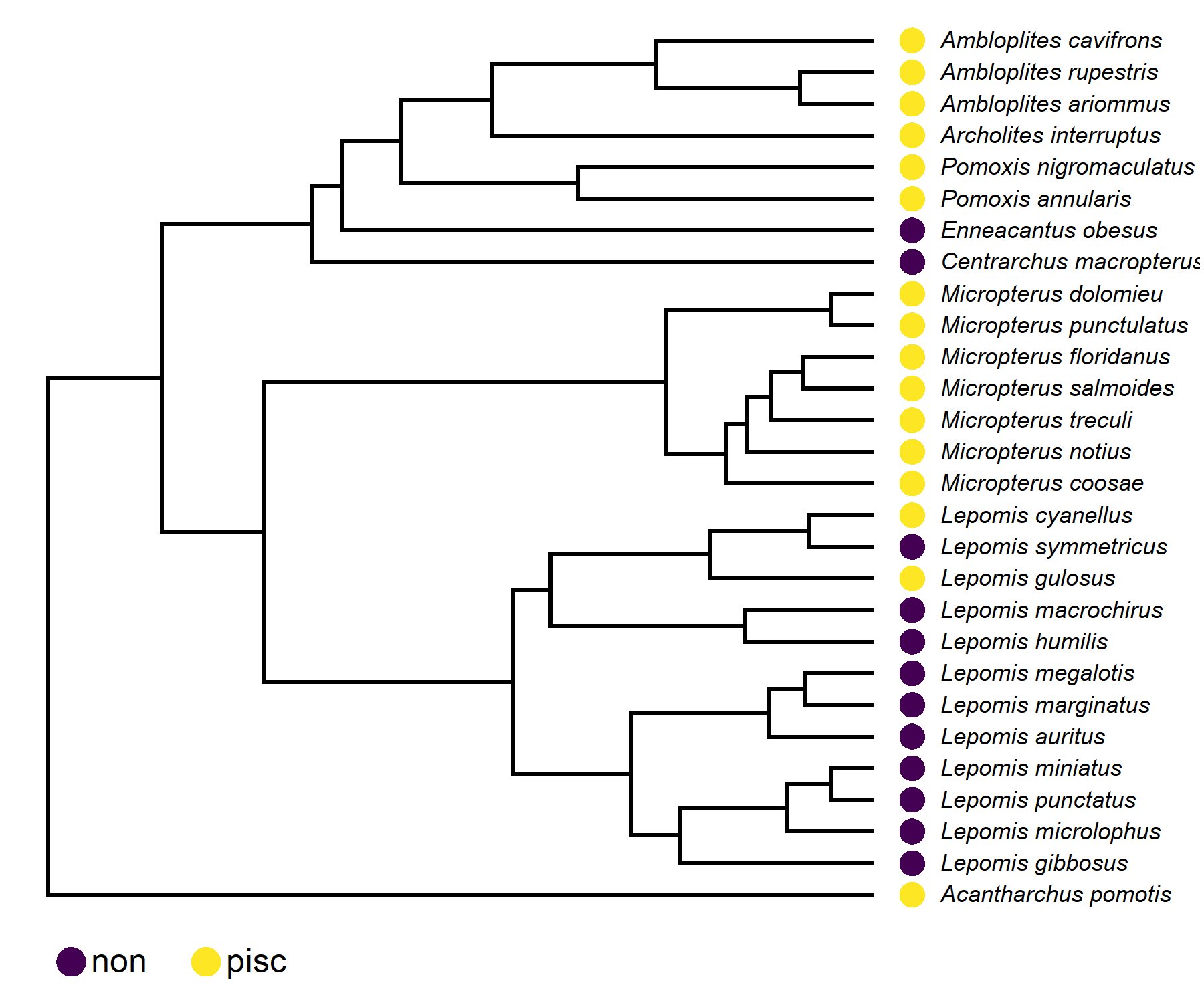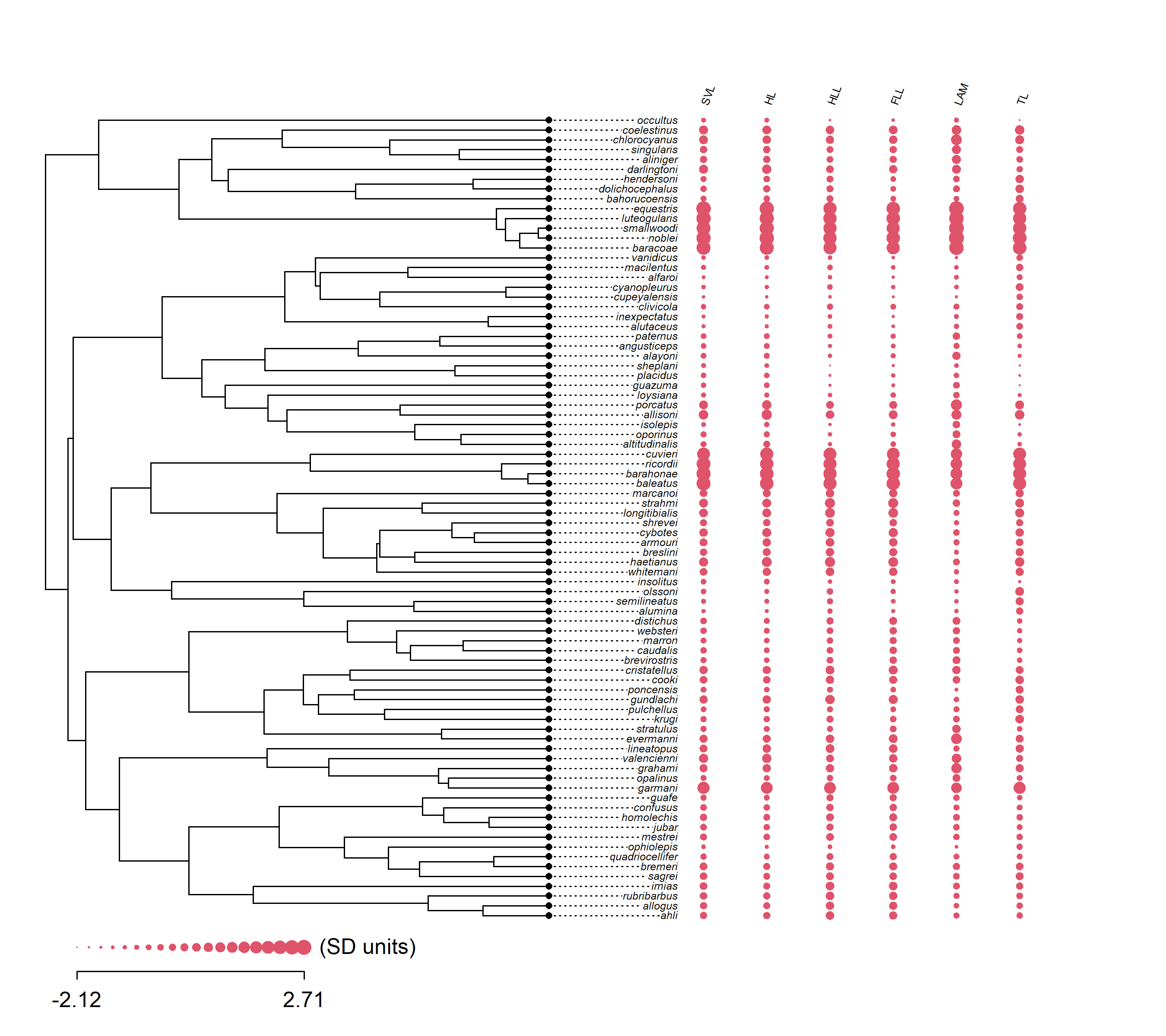## Monday, June 5, 2023

### Control dot border colors in phytools`dotTree`

A phytools user recently asked if it was possible to control the outline color of dots in the the `dotTree` function for plotting discrete or continuous character data as dots adjacent to the tips of the phylogeny.

The answer was no; however, I have now pushed an update to allow this, which can be obtained by updating phytools from GitHub.

Here’s a very quick demo.

``````library(phytools)
``````

``````data(sunfish.data)
data(sunfish.tree)
``````

Create the plot using default border settings.

``````cols<-setNames(viridisLite::viridis(n=2),
levels(sunfish.data[]))
dotTree(sunfish.tree,sunfish.data[,1,drop=FALSE],ftype="i",
colors=cols,fsize=0.7)
``````OK, now let’s make some adjustments based on the new options available to us.

``````dotTree(sunfish.tree,sunfish.data[,1,drop=FALSE],ftype="i",
colors=cols,fsize=0.7,border="blue")
``````More commonly, I imagine, users might like to do away with the border altogether. This can be accomplished by setting it to `"transparent"` (or to the background color of the plot, for that matter) as follows. Note that in this case the plotted size of our dots will seem to shrink, so I added the function argument `cex.dot` to account for that.

``````dotTree(sunfish.tree,sunfish.data[,1,drop=FALSE],ftype="i",
colors=cols,fsize=0.7,border="transparent",cex.dot=1.1)
``````Excellent!

The same set of arguments also works for continuous character data. For example:

``````data("anoletree")
data("anole.data")
dotTree(anoletree,anole.data,fsize=0.5,border="transparent",cex.dot=1.5,
length=20,standardize=TRUE,colors=palette(),labels=TRUE)
``````That’s all for now.